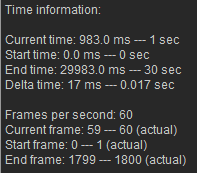# IC Python API:Managing Time

Main article: RL Python Samples.

Managing time is crucial for dealing with animation, and understanding what happens under the hood is of utmost importance for scripting animated effects. This article will describe the ways to handle time in a way that is relevant and useful for coding.

## Required Modules

We'll need to access the main Reallusion Python module to work with global and time elements.

```import RLPy
```

## Important Time Elements

RGlobal provides import information on the current project timeline among many other useful data.

```# Retrieve time attributes
current_time = RLPy.RGlobal.GetTime()  # Position of the playhead
start_time = RLPy.RGlobal.GetStartTime()  # Position of the mark-in flag
end_time = RLPy.RGlobal.GetEndTime()  # Position of the mark-out flag
```

## Important Frame Elements

In order to get frame based timeline data, we must derive them with a combination of RGlobal and RTime.

```# Retrieve frame attributes
fps = RLPy.RGlobal.GetFps()  # Native frame rate for iClone is 60 fps
current_frame = RLPy.RTime.GetFrameIndex(current_time, fps)  # Position of the playhead
start_frame = RLPy.RTime.GetFrameIndex(start_time, fps)  # Position of the mark-in flag
end_frame = RLPy.RTime.GetFrameIndex(end_time, fps)  # Position of the mark-out flag
```

## Other Useful Information

You might also need other important time data such as delta-time which represents the time passed after the completion of the last frame. You might use this to sync animation with the current project fps.

```# Other useful information
delta_time = 1 / fps  # Time in seconds for each frame
```

## Displaying EverythingTimeline units are always placed 1 frame behind the code derived values.

Let's make a fancy print of the information gathered thus far.

```print(f'''Time information:

Current time: {current_time.GetValue()} ms --- {round(current_time.GetValue()/1000)} sec
Start time: {start_time.GetValue()} ms --- {round(start_time.GetValue()/1000)} sec
End time: {end_time.GetValue()} ms --- {round(end_time.GetValue()/1000)} sec
Delta time: {round(delta_time * 1000)} ms --- {round(delta_time, 3)} sec

Frames per second: {fps}
Current frame: {current_frame} --- {current_frame+1} (actual)
Start frame: {start_frame} --- {start_frame+1} (actual)
End frame: {end_frame} --- {end_frame+1} (actual)
```

## Everything Put Together

You can copy and paste the following code into a PY file and load it into iClone via Script > Load Python.

```import RLPy

# Retrieve time attributes
current_time = RLPy.RGlobal.GetTime()  # Position of the playhead
start_time = RLPy.RGlobal.GetStartTime()  # Position of the mark-in flag
end_time = RLPy.RGlobal.GetEndTime()  # Position of the mark-out flag

# Retrieve frame attributes
fps = RLPy.RGlobal.GetFps()  # Native frame rate for iClone is 60 fps
current_frame = RLPy.RTime.GetFrameIndex(current_time, fps)  # Position of the playhead
start_frame = RLPy.RTime.GetFrameIndex(start_time, fps)  # Position of the mark-in flag
end_frame = RLPy.RTime.GetFrameIndex(end_time, fps)  # Position of the mark-out flag

# Other useful information
delta_time = 1 / fps  # Time in seconds for each frame

print(f'''Time information:

Current time: {current_time.GetValue()} ms --- {round(current_time.GetValue()/1000)} sec
Start time: {start_time.GetValue()} ms --- {round(start_time.GetValue()/1000)} sec
End time: {end_time.GetValue()} ms --- {round(end_time.GetValue()/1000)} sec
Delta time: {round(delta_time * 1000)} ms --- {round(delta_time, 3)} sec

Frames per second: {fps}
Current frame: {current_frame} --- {current_frame+1} (actual)
Start frame: {start_frame} --- {start_frame+1} (actual)
End frame: {end_frame} --- {end_frame+1} (actual)
''')
```

### APIs Used

You can research the following references for the APIs deployed in this code.